SEISMIC ATTRIBUTES BASICS
Seismic petrophysics, at its best, tries to quantify reservoir properties from seismic data instead of from well logs. Sophisticated data processing with high quality seismic data can come close to achieving this, at least in some cases.  The process is called seismic inversion and the results are often called seismic attributes. These atributes are closely related to the mechanical properties (elastic constants) of the rocks, which in turn can be transformed into reservoir properties of interest, such as porosity, lithology, or fluid type.

The process is sometimes called "quantitative seismic interpretation". In high porosity areas such as the tar sands, and in high contrast areas such as gas filled carbonates, modest success has been achieved, usually after several iterative calibrations to log and lab data. Something can be determined in almost all reservoirs, but how "quantitative" it is may not be known. Semi-quantitative or qualitative attribute presentations are often sufficient to create a reasonable image of the reservoir.

Today, seismic processing and well log analysis are combined to determine seismic attributes. The vertical resolution of seismic data is far less than that of well logs, so some filtering and up-scaling issues have to be addressed to make the comparisons meaningful.

There are many other types of seismic attributes, related to the signal frequency, amplitude, and phase, as well as spatial attributes that infer geological structure and stratigraphy, such as dip angle, dip azimuth, continuity, thickness, and a hundred other factors. While logs may be used to calibrate or interpret some of these attributes, they are not discussed further here.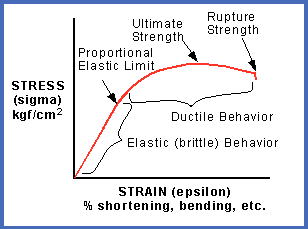The best known elastic constants are the bulk modulus of compressibility, shear modulus, Young's Modulus (elastic modulus), and Poisson's Ratio. The dynamic elastic constants can be derived with appropriate equations, using sonic log compressional and shear travel time along with density log data. Specific forms of seismic inversion can approximate the log analysis res

Elasticity is a property of matter, which causes it to resist deformation in volume or shape. Hooke's Law, describing the behavior of elastic materials, states that within elastic limits, the resulting strain is proportional to the applied stress. Stress is the external force applied per unit area (pressure), and strain is the fractional distortion which results because of the acting force.

The modulus of elasticity is the ratio of stress to strain:
0: M = Pressure / Change in Length =  {F/A} / (dL/L)

This is identical to the definition of Young's Modulus. Both names are used in the literature so terminology can be a bit confusing.Different types of deformation can result, depending upon the mode of the acting force. The three elastic moduli are:

Young's Modulus Y (also abbreviated E in various literature),
1: Y = (F/A) / (dL/L)

Bulk Modulus Kc,
2: Kc = (F/A) / (dV/V)

Shear Modulus N, (also abbreviated as u (mu))
3: N = (F/A) / (dX/L) = (F/A) / tanX

Where F/A is the force per unit area and dL/L, dV/V, and (dX/L) = tanX are the fractional strains of length, volume, and shape, respectively.Poisson's Ratio PR (also abbreviated v (nu)),  defined as the ratio of strain in a perpendicular direction to the strain in the direction of extensional force,
4: PR = (dX/X) / (dY/Y)

Where X and Y are the original dimensions, and dX and dY are the changes in x and y directions respectively, as the deforming stress acts in y direction.

Young's Modulus vs Poison's Ratio: Brittleness increases toward top left, density increases toward top right, porosity plus organic content and depth decrease toward bottom left. PR values less than 0.17 indicate gas or organic content or both. (image courtesy Canadian Discovery Ltd)

All of these moduli can be derived directly from well logs and indirectly from seismic attributes:
5: N = KS5 * DENS / (DTS ^ 2)
6: R = DTS / DTC
7: PR = (0.5 * R^2 - 1) / (R^2 - 1)
8: Kb = KS5 * DENS *(1 / (DTC^2) - 4/3 * (1 / (DTS^2)))
9: Y = 2 * N * (1 + PR)

Lame's Constant Lambda, (also abbreviated λ) is a measure of a rocks brittleness, which is a function of both Young's Modulus and Poisson's Ratio:
10:
Lambda = Y * PR / ((1 + PR) * (1 - 2 * PR))
OR 10A: Lambda = DENS * (Vp^2 - 2 * Vs ^ 2)

Some people prefer different abbreviations: Mu or u for shear modulus, Nu or v for Poisson's Ratio, and E for Young's Modulus. The abbreviations used above are used consistently trough these training materials.

In the seismic industry, it is common to think in terms of velocity and acoustic impedance in addition to the more classical mechanical properties described above.

The compressional to shear velocity ratio is a good lithology indicator:
11. R = Vp / Vs = DTS / DTC

Acoustic impedance:
12: Zp = DENS / DTC
13: Zs = DENS / DTS

Where:
DTC = compressional sonic travel time
DTS = shear sonic travel time
DENS = bulk density
KS5 = 1000 for metric units

An example of a log analysis for mechanical rock properties (elastic constants) is shown below. Coloured dots represent lab derived data, and illustrate the close match obtained betwee log analysis and lab measured data.Dynamic elastic properties calculated from density and sonic log data, showing close match to dynamic data from lab measurements (coloured dots). Lab data is from table shown above. Note synthetic sonic and density plotted next to measured log curves (Tracks 2 and 3), showing reasonably small differences due to minor borehole effects. Synthetic curves can repair worse logs or even replace missing curves.

Composite seismic attributes, such as Lame's Constant times density (Lambda_Rho) and shear modulus times density (Mu_Rho), are used to normalize attributes to make interpretation easier. Various crossplots of results are used to distinguish differences between rock types, as shown below. The colour code represents depth (red-orange = shallower, blue-green = deeper)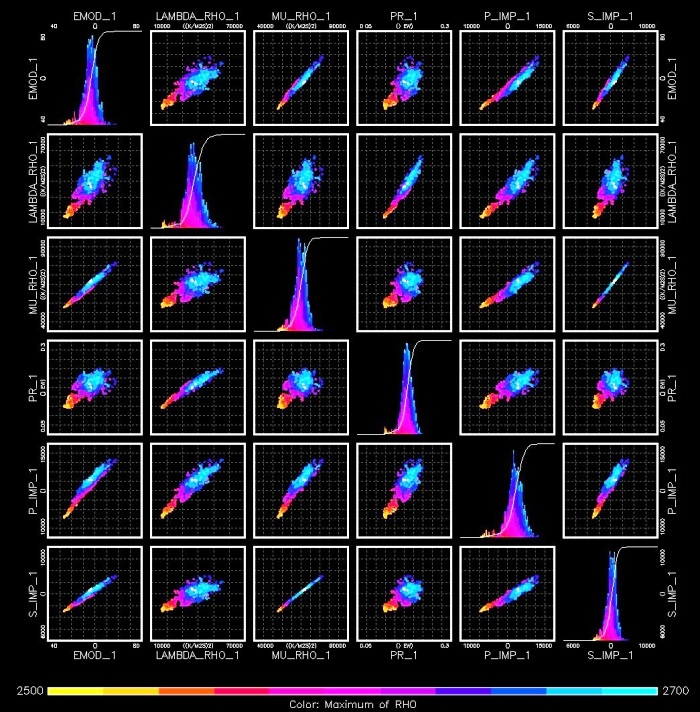Crossplots of the elastic constants are used to identify variations in rock characteristics, by noting changes in the data distributions. (RHO = density, PR = Poisson's Ratio, MU = shear Modulus, LAMBDA = Lame's Constant, BMOD = bulk modulus, EMOD = Young's Modulus, P_IMP = compressional wave acoustic impedance, S_IMP = shear wave acoustic impedance, (image courtesy Canadian Discover Ltd)

The current capability of seismic inversion has made significant progress in the accuracy of seismic attributes and the interpretation of the results. The following example is from "Quantitative Seismic Interpretation in the Canadian Oil Sands" by Laurie Weston Bellman, Dubai, 2012.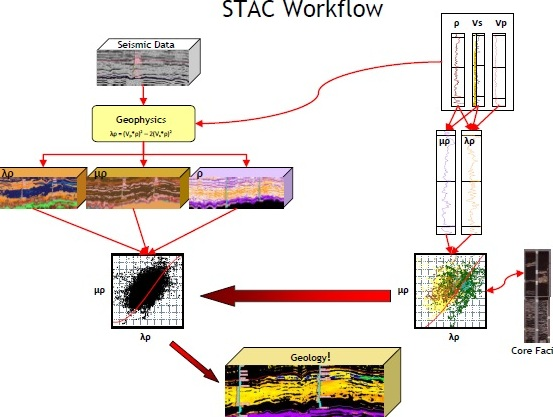Illustrated workflow for a quantitative seismic inversion for lithology and fluid type. STime domain seismic data is inverted into ccompressional, shear, and Lambda domains. Well log data is transformed into the same parameters and crossplotted. These crossplots are used to limit similar crossplots derived from the iseismic inversions. The final prodoct is an inversion showings sand with bitumen (light colour), bottom water (blue), top gas (green) and shale (black).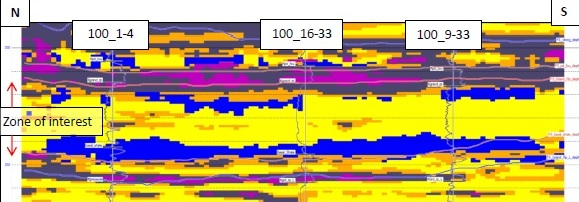Enlarged detail of quantitative seismic inversion - colours same as previous example.ELASTIC CONSTANTS THEORY
The velocity of sound in a rock is related to the elastic properties of the rock/fluid mixture and its density, according to the Wood, Biot, and Gassmann equations.

The composite compressional bulk modulus of fluid in the pores (inverse of fluid compressibility) is:   ____
1:  Kf = 1/Cf = Sw / Cwtr + (1 - Sw) / Coil
OR 1a: Kf = 1/Cf = Sw / Cwtr + (1 - Sw) / Cgas

The pore space bulk modulus (Kp) is derived from the porosity, fluid, and matrix rock properties:
2: ALPHA = 1 - Kb / Km
3: Kp = ALPHA^2 / ((ALPHA - PHIt) / PHIt / Kf )

The composite rock/fluid compressional bulk modulus is:
4: Kc = Kp + Kb + 4/3 * N

Compressional and shear velocity (or travel time) depend on density and on the elastic properties, so we need a density value that reflects the actual composition of the rock fluid mixture:
5:  DENS = (1 - Vsh) * (PHIe * Sw * DENSW + PHIe * (1 - Sw) * DENSHY + (1 - PHIe) * DENSMA)
+ Vsh * DENSSH

Compressional velocity (Vp) and shear velocity (Vs) are defined as:
6: Vp = KS4 * (Kc / DENS) ^ 0.5
7: Vs = KS4 * (N / DENS) ^ 0.5

Although it is not a precise solution, we often invert equations 5 and 6 to solve for Kb and N from sonic log compressional and shear travel time values.

Where:
ALPHA = Biot's elastic parameter (fractional)
Cgas = gas compressibility (1/GPa)
Coil = oil compressibility (1/GPa)
Cwtr = water compressibility (1/GPa)
DENS = rock density (g/cc)
DENSW = density of fluid in the pores (g/cc)
DTC = compressional sonic travel time (usec/m)
DTS = shear sonic travel time (usec/m)
Kb = compressional bulk modulus of empty rock frame (GPa)
Kc = compressional bulk modulus of porous rock (GPa)
Kf = compressional bulk modulus of fluid in the pores (GPa)
Km = compressional bulk modulus of rock grains (GPa)
Kp = compressional bulk modulus of pore space (GPa)
N = shear modulus of empty rock frame (GPa)
PHIt = total porosity of the rock (fractional)
Sw = water saturation (fractional)
Vp = compressional wave velocity (m/sec)
Vs = shear wave velocity (m/sec)
Vp = Stoneley wave velocity (m/sec)
KS4 = 1000 for semi-Metric units shown above - convert kg/m3 to g/cc

The Biot-Gassmann approach looks deceptively simple. However, the major drawback to this approach is the difficulty in determining the bulk moduli, particularly those of the empty rock frame (Kb and N), which cannot be derived from log data. Murphy (1991) provided equations for sandstone rocks (PHIe < 0.35) that predict Kb and N from porosity:
8: Kb = 38.18 * (1 - 3.39 * PHIe + 1.95 * PHIe^2)
9: N   = 42.65 * (1 - 3.48 * PHIe + 2.19 * PHIe^2)

 RECOMMENDED PARAMETERS: Water Salinity Cf psi-1 Kf psi Cf GPa-1 Kf GPa 5000 0.0000040 250000 0.580 1.723 35000 0.0000039 270270 0.537 1.862 200000 0.0000027 344828 0.420 2.376 Oil Depth 2000 ft 610 m 0.0000085 117647 1.233 0.811 4000 ft 1220 m 0.0000095 105263 1.378 0.725 8000 ft 2440 m 0.0000116 86207 1.683 0.594 12000 ft 3660 m 0.0000135 74074 1.959 0.510 Gas Depth 2000 ft 610 m 0.001250 800 181.422 0.006 4000 ft 1220 m 0.000510 1961 74.020 0.014 8000 ft 2440 m 0.000180 5556 26.124 0.038 12000 ft 3660 m 0.000100 10000 14.513 0.069Examples of Mechanical Properties Logs
The format and curve complement of Mechanical Properties Logs vary widely between service companies and age of log. Some logs have Metric depths but the moduli are in English units. Some are vice versa. Here are some examples.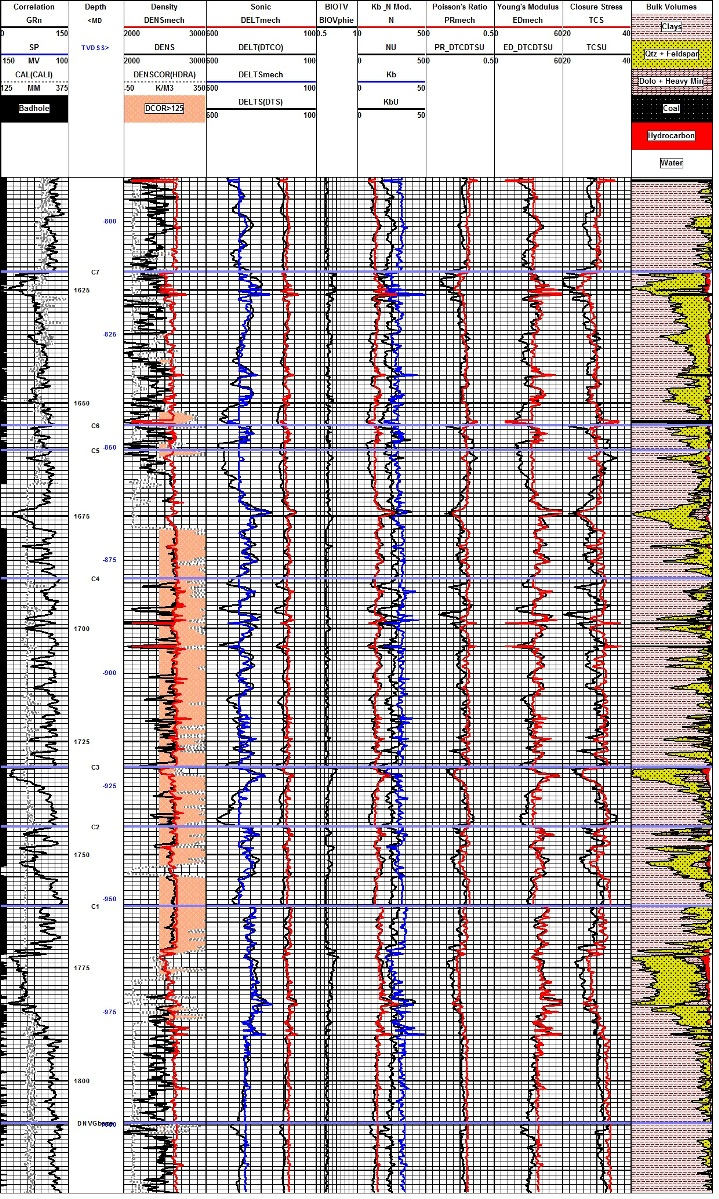Example of log reconstruction in a shaly sand sequence (Dunvegan). The 3 tracks on the left show the measured gamma ray, caliper, density, and compressional sonic. Original density and sonic are shown in black, modeled logs are in colour. Shear sonic is the model result as none was recorded in this well. Computed elastic properties are shown in the right hand tracks. Results from the original unedited curves are shown in black, those after log editing are in colour. Note that the small differences in the modeled logs compared to the original curves propagate into larger differences in the results, especially Poisson's Ratio (PR), Young's Modulus (ED), and total closure stress (TCS).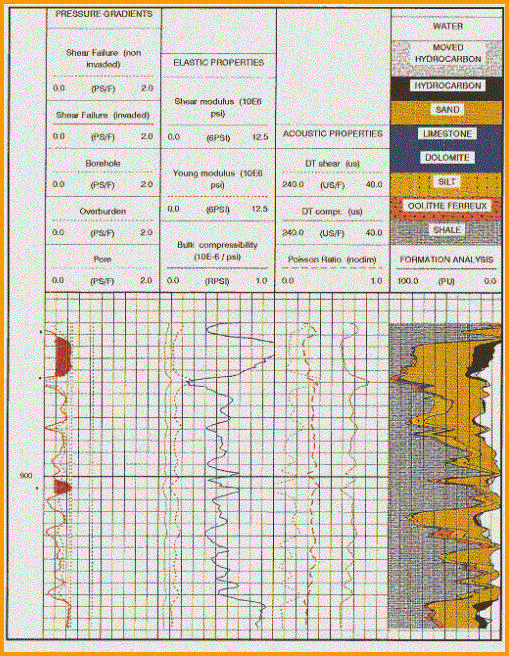Mechanical properties log with lithology/porosity track at the right. This analysis was run to find out if sanding might occur during production from the oil zone. High bulk modulus and low sher modulus suggest sanding is like. Stress failre (shaded black in Track 1) shows where sanding is most likely to occur.

Page Views ---- Since 01 Jan 2015
Copyright 2023 by Accessible Petrophysics Ltd.
CPH Logo, "CPH", "CPH Gold Member", "CPH Platinum Member", "Crain's Rules", "Meta/Log", "Computer-Ready-Math", "Petro/Fusion Scripts" are Trademarks of the Author